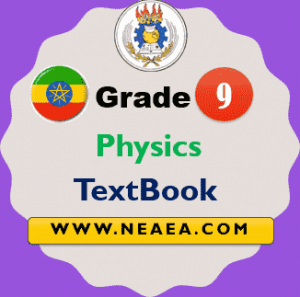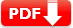# Ethiopian Grade 9 Physics Textbook [PDF] For Students

Ethiopian Grade 9 Physics Textbook [PDF] For Students. It is provided by the MoE Ethiopia for free. This textbook is based on the new Curriculum Framework for Ethiopian Education. Students, parents, and teachers can download the same from the Federal Democratic Republic of Ethiopia Ministry of Education official website.

The Physics content of Grades 9 and 10 is a continuation of topics met in Grades 7 and 8 with deepening and broadening further and adding new topics. At this level, the emphasis is upon a deeper understanding of underlying concepts involving measurement, equations, and simple calculations.## Ethiopian Grade 9 Physics Student textbook

Grades 9 and 10 are categorized as the first cycle of secondary education. This is due to the fact that the students will be streamed after finishing Grade 10, which is a terminal point. This fact is given due consideration in preparing this curriculum guide. Every effort has been made to make the citizens which complete the first cycle of the secondary education knowledgeable of the most general basic laws of physics which they will apply in their daily lives.

Unit 1: Vectors

• 1.1. Representation of vectors
• 1.2. Addition and subtraction of vectors
• 1.3. Some applications of vectors

Unit 2: Motion in a straight line

• 2.1. Uniform motion
• 2.2. Uniformly accelerated motion
• 2.3. Graphical description of uniformly accelerated motion
• 2.4. Equations of uniformly accelerated motion
• 2.5. Relative velocity in one dimension

Unit 3: Force and Newton’s laws of motion

• 3.1. Forces in nature
• 3.2. Newton’s 2nd law
• 3.3. Frictional force
• 3.4. Newton’s 3rd law
• 3.5. Conservation of linear momentum
• 3.6. Collisions
• 3.7. The first condition of equilibrium

Unit 4: Work, energy, and power

• 4.1. Mechanical work
• 4.2. Work-energy theorem
• 4.3. Conservation of energy
• 4.4. Mechanical power

Unit 5: Simple machines

• 5.1. Purposes of machines
• 5.2. Inclined plane, wedge, and screw
• 5.3. Levers

Unit 6: Fluid statics

• 6.1. Air pressure
• 6.2. Fluid pressure

Unit 7: Temperature and heat

• 7.1. Temperature and heat energy
• 7.2. Expansion of solids, liquids and gases
• 7.3. Quantity of heat, specific heat capacity, and heat capacity
• 7.4. Change of state

Unit 8: Wave motion and sound

• 8.1. Wave propagation
• 8.2. Mechanical waves
• 8.3. Properties of waves
• 8.4. Sound waves

 Country Ethiopia Publisher MoE, Ethiopia Class Grade 9 Subject Grade 9 Physics Type TextBook Group General System Total Units 8 Total Pages 250 TextBook Version 1.0 Download Link